# PMSM Constraint Curves and Their Application

This example uses Motor Control Blockset™ to explain the fundamentals of constraint curves, untilization of these curves to determine operating currents, and usage of the grid of these currents in simulation or deployment environments.

The first section Fundamentals of Constraint Curves of PMSM explains about the constraint curves of a Permanent Magnet Synchronous Machine (PMSM). The five curves (current limit circle, constant torque curve, MTPA curve, voltage limit curve, and MTPV curve) represent the constraints and characteristics of the motor system.

The second section Using Constraint Curves explores the applications of the constraint curves in identifying the optimal operating points to find reference currents in d- and q-axes for a given operating speed and reference torque. The section shows how to create Look-up tables (LUT) of reference currents. You will come across a group of five operating points across different regions, which the example elaborates.

The third section Identifying Possible (MTPA-Field Weakening-MTPV) Control Currents shows how to use the LUT based PMSM Control Reference block to find the operating points in a simulation environment.

### Required MathWorks® Products

• Motor Control Blockset

### Fundamentals of Constraint Curves of PMSM

Constraint curves represent the operational constraints of the PMSM control. Each curve corresponds to either a constraint or a characteristic behavior.

The following code can be used to re-create figure 1.

```%{ pmsm=mcb_SetPMSMMotorParameters('Teknic2310P'); pmsm.Rs=0.01; pmsm.Lq=pmsm.Ld*2; inverter=mcb_SetInverterParameters('BoostXL-DRV8305'); mcbPMSMCharacteristics(pmsm,inverter,speed=5500,torque=0.1394); %}```Figure 1: Representation of constraint curves of a PMSM

The d-q Frame of Reference

The d-q frame of reference is a rotating frame of reference, that moves along with the rotor. This frame of reference, as opposed to the stationary frame of reference, simplifies the representation of motor's electrical quantities, such as voltages and currents.

You can measure the motor phase currents ia, ib and ic and transform them into d-q reference frame currents (id, iq) using the knowledge of rotor position. For a balanced three phase system, you can assume the zero-sequence current to be zero. For more details about the Clarke and Park transformations, see Clarke and Park Transforms - MATLAB & Simulink (mathworks.com).

The d-q Current Space

This is the imaginary frame of reference with the d-axis current represented in the x-axis, and the q-axis current represented in the y-axis.

You can plot a given PMSM's limits and characteristics (when represented in terms of id and iq) in the d-q current space.

### Current Limit Circle

This is the operating limit of the system on the current. Because the net current of the system is limited as well as the id and iq components are orthogonal, these currents are related in the following manner:

`${i}_{d}^{2}+{i}_{q}^{2}={i}_{s}^{2}\le {i}_{s_\mathrm{max}}^{2}$`

where,

• ${i}_{d}$ is the d-axis current (in Amperes).

• ${i}_{q}$ is the q-axis current (in Amperes).

• ${i}_{s}$ is the source current (in Amperes).

• ${i}_{s_\mathrm{max}}$ is the maximum source current (in Amperes).

Because is_max is constant for a given constraint, locus of all the points that satisfy this relation represents a circle.

Any point inside this circle (id, iq) has a magnitude that is less than the current limit is_max. Therefore, all the points inside the circle are valid points when considering the current constraint.

Irrespective of the motor topology (SPMSM or IPMSM), the current limit circle remains a circle.

The following figure shows the plot of a current limit circle in black color with an is_max of 7.1A.Figure 2: A current limit circle or current limit curve of a PMSM

### Constant Torque Curve

Constant torque curve is a locus of all (id,iq) points that deliver a same torque.

The torque of a PMSM includes reluctance torque and PM torque. The following equation represents the generated torque:

`$T=1.5\phantom{\rule{0.2222222222222222em}{0ex}}polePairs\phantom{\rule{0.2222222222222222em}{0ex}}\left({i}_{q}\phantom{\rule{0.2222222222222222em}{0ex}}FluxPM+\left({L}_{d}-{L}_{q}\right)\phantom{\rule{0.2222222222222222em}{0ex}}{i}_{d}\phantom{\rule{0.2222222222222222em}{0ex}}{i}_{q}\right)$`

where,

• $polePairs$ is the number of pole pairs in the PMSM.

• $FluxPM$ is the permanent magnet flux linkage (Weber).

• ${i}_{d}$ and ${i}_{q}$ are the d-axis and q-axis currents respectively (in Amperes).

• ${L}_{d}$ and ${L}_{q}$ are the d-axis and q-axis inductances respectively (in Henry).

For a given fixed torque, the preceding equation transforms to ${i}_{q}=\frac{{K}_{1}}{{K}_{2}+{i}_{d}}$, which forms a hyperbola representation.

Any (id, iq) value pair on the constant torque curve represents currents that satisfy the load torque. To ensure that (id,iq) also satisfy the constraint, you can check if this point satisfies the current constraint (inside the closed circle).Figure 3: Current limit circle and constant torque curve of PMSM.Figure 4: Current limit circle and a group of constant torque curves drawn at different torque values in an increasing order. The lighter shade of the constant torque curve represents an increasing torque.

### MTPA Curve

MTPA stands for Maximum Torque Per Ampere. An MTPA curve is a locus of all points that satisfy the optimization condition providing a maximum torque for a given current.

You can obtain the expression for MTPA curve (using linear motor parameters) with an assumption that the partial derivative of the torque with respect to current (either id or iq) is equal to zero.

For an IPMSM, the following expression represents an MTPA trajectory:

`${i}_{d}^{2}+\frac{{i}_{d}\phantom{\rule{0.2222222222222222em}{0ex}}FluxPM}{{L}_{d}-{L}_{q}}={i}_{q}^{2}$`

where,

• $FluxPM$ is the permanent magnet flux linkage (Weber).

• ${i}_{d}$ and ${i}_{q}$ are the d-axis and q-axis currents respectively (in Amperes).

• ${L}_{d}$ and ${L}_{q}$ are the d-axis and q-axis inductances respectively (in Henry).

The preceding equation represents a parabola. All points on the MTPA curve satisfy the condition that they provide maximum torque per current.Figure 5: Current limit circle, a group of constant torque curves, and a smaller current limit circle representing the current limit for one of the constant torque curves.

When we consider the operating current as a virtual current limit for a hypothetical system and draw the constraint curves including a constant torque curve and a current limit circle, they both intersect tangentially at a single point. This point represents the maximum possible torque that you can achieve using the virtual current limit for the hypothetical system.Figure 6: A group of pairs of current limit circles with different limit values and the corresponding maximum torques.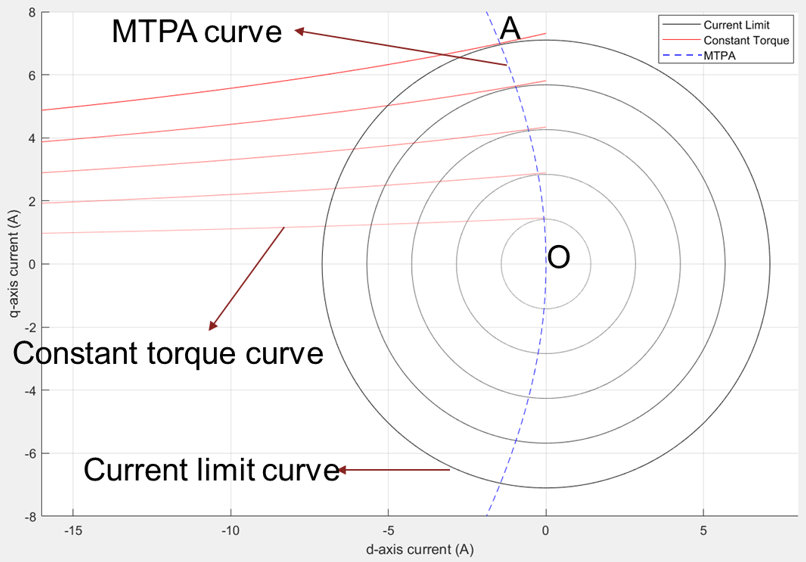Figure 7: A group of pairs of current limit circles with different limit values with the corresponding maximum torques and the MTPA curve.

As shown in figure 7, the MTPA curve is a collection of all points which are intersecting points of current limit circles and the corresponding constant torque curves (drawn at maximum possible torque for the given current). This curve represents visually how MTPA can be understood in the form of points representing the maximum torque for a given current.

### Voltage Limit Curve

Voltage limit curve is the locus of all (id,iq) points for a specified speed and voltage, which result in the system voltage, $\sqrt{{v}_{d}^{2}+{v}_{q}^{2}}$, reaching the voltage limit, vmax.

The following equations represent the phase voltages in the d-q reference frame:

${v}_{d}={i}_{d}\phantom{\rule{0.2222222222222222em}{0ex}}{R}_{s}-w\phantom{\rule{0.2222222222222222em}{0ex}}FluxQ$, where $FluxQ={L}_{q}\phantom{\rule{0.2222222222222222em}{0ex}}{i}_{q}$

${v}_{q}={i}_{q}\phantom{\rule{0.2222222222222222em}{0ex}}{R}_{s}+w\phantom{\rule{0.2222222222222222em}{0ex}}FluxD$, where $FluxD={L}_{d}\phantom{\rule{0.2222222222222222em}{0ex}}{i}_{d}+FluxPM$

where,

• $FluxD$ and $FluxQ$ are the d-axis and q-axis flux linkages respectively (in Weber).

• $FluxPM$ is the permanent magnet flux linkage (Weber).

• ${i}_{d}$ and ${i}_{q}$ are the d-axis and q-axis currents respectively (in Amperes).

• ${v}_{d}$ and ${v}_{q}$ are the d-axis and q-axis voltages respectively (in Volts).

• ${L}_{d}$ and ${L}_{q}$ are the d-axis and q-axis inductances respectively (in Henry).

• $w$ is the rotor speed (in Radians/ sec).

• ${R}_{s}$ is the stator phase winding resistance (in Ohms).

The system voltage is constrained by the operating power supply voltage and the PWM scheme. For SVPWM, the available voltage is ${v}_{\mathrm{max}}=\frac{{V}_{dc}}{\sqrt{3}}$, while for Sine PWM, the available voltage is ${v}_{\mathrm{max}}=\frac{{V}_{dc}}{2}$.

`${v}_{d}^{2}+{v}_{q}^{2}={v}_{s}^{2}\le {v}_{\mathrm{max}}^{2}$`

where,

• ${v}_{d}$ and ${v}_{q}$ are the d-axis and q-axis voltages respectively (in Volts).

• ${v}_{s}$ is the source voltage (in Volts).

• ${v}_{\mathrm{max}}$ is the maximum fundamental line to neutral voltage (peak) supplied to the motor (in Volts).

The system voltage vs is equal to or less than vmax to satisfy the voltage constraint.

After inserting vd and vq representations in the preceding equation, you get:

`${\left({i}_{d}\phantom{\rule{0.2222222222222222em}{0ex}}{R}_{s}-w\phantom{\rule{0.2222222222222222em}{0ex}}{L}_{q}\phantom{\rule{0.2222222222222222em}{0ex}}{i}_{q}\right)}^{2}+{\left({i}_{q}\phantom{\rule{0.2222222222222222em}{0ex}}{R}_{s}+w\phantom{\rule{0.2222222222222222em}{0ex}}{L}_{d}\phantom{\rule{0.2222222222222222em}{0ex}}{i}_{d}+w\phantom{\rule{0.2222222222222222em}{0ex}}FluxPM\right)}^{2}={v}_{s}^{2}\le {v}_{\mathrm{max}}^{2}$`

where,

• $FluxPM$ is the permanent magnet flux linkage (Weber).

• ${i}_{d}$ and ${i}_{q}$ are the d-axis and q-axis currents respectively (in Amperes).

• ${v}_{d}$ and ${v}_{q}$ are the d-axis and q-axis voltages respectively (in Volts).

• ${v}_{s}$ is the source voltage (in Volts).

• ${v}_{\mathrm{max}}$ is the maximum fundamental line to neutral voltage (peak) supplied to the motor (in Volts).

• ${L}_{d}$ and ${L}_{q}$ are the d-axis and q-axis inductances respectively (in Henry).

• $w$ is the rotor speed (in Radians/ sec).

• ${R}_{s}$ is the stator phase winding resistance (in Ohms).

This equation represents a generalized ellipse.

SPMSM has Ld=Lq, while for IPMSM, Lq>Ld, where Ld and Lq are inductances along the d- and q-axes respectively.

Due to the above parameter variation, the voltage limit curve of the SPMSM is a circle, with a center away from the origin. The shift from the origin (id=0, iq=0) depends on the motor system parameters FluxPM, speed, Rs, Ld, and Lq.

Because for an IPMSM, Ld and Lq are not identical, the generated voltage limit curve is an ellipse.Figure 8: Current limit circle, voltage limit curve, and MTPA curve of a PMSM.

Figure 8 includes the voltage limit ellipse for a given motor. You can see that the current limit circle is centered around the origin and the voltage limit ellipse is not centered around the origin. The region of overlap between the voltage limit ellipse and the current limit circle represent the possible (id, iq) current values that satisfy both the voltage and current constraints.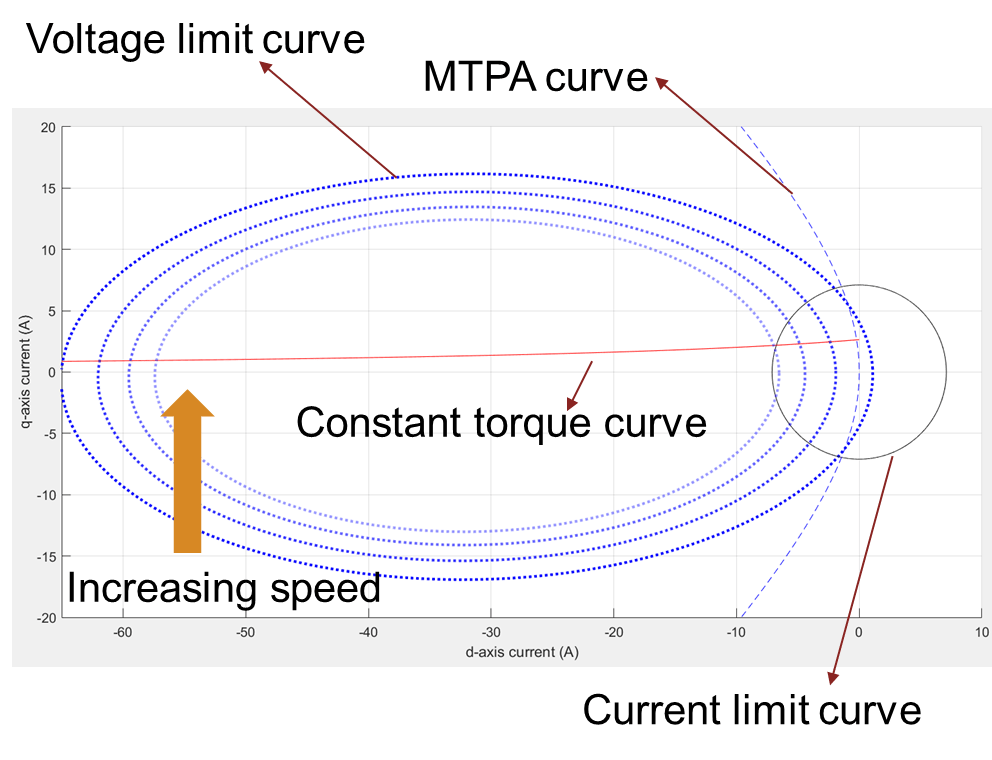Figure 9: Current limit circle, MTPA curve, and a group of voltage limit curves drawn at different speeds. The lighter dotted blue lines represent the increasing direction of speed.

Voltage Limit Curve - Approximate Equations vs Actual Equations

A simpler form of the voltage limit curve is called the approximate representation. In this representation, you can ignore the $i\phantom{\rule{0.2222222222222222em}{0ex}}r$ component in the vd and vq equations and compensate them in the vmax term.

In the approximate representation, the following equations represent the phase voltages in the d-q reference frame:

${v}_{d}=-w\phantom{\rule{0.2222222222222222em}{0ex}}FluxQ$, where $FluxQ={L}_{q}\phantom{\rule{0.2222222222222222em}{0ex}}{i}_{q}$

${v}_{q}=w\phantom{\rule{0.2222222222222222em}{0ex}}FluxD$, where $FluxD={L}_{d}\phantom{\rule{0.2222222222222222em}{0ex}}{i}_{d}+FluxPM$

The system voltage is constrained by the operating power supply voltage and the PWM scheme. For SVPWM, the available voltage is ${v}_{\mathrm{max}}=\frac{{V}_{dc}}{\sqrt{3}}-{i}_{\mathrm{max}}\phantom{\rule{0.2222222222222222em}{0ex}}{R}_{s}$, while for Sine PWM, the available voltage is ${v}_{\mathrm{max}}=\frac{{V}_{dc}}{2}-{i}_{\mathrm{max}}\phantom{\rule{0.2222222222222222em}{0ex}}{R}_{s}$.

`${v}_{d}^{2}+{v}_{q}^{2}={v}_{s}^{2}\le {v}_{\mathrm{max}}^{2}$`

The system voltage vs that is equal to or less than vmax satisfies the voltage constraint.

After inserting vd and vq representations in the preceding equation, you get:

`${\left(-w\phantom{\rule{0.2222222222222222em}{0ex}}{L}_{q}\phantom{\rule{0.2222222222222222em}{0ex}}{i}_{q}\right)}^{2}+{\left(w\phantom{\rule{0.2222222222222222em}{0ex}}{L}_{d}\phantom{\rule{0.2222222222222222em}{0ex}}{i}_{d}+w\phantom{\rule{0.2222222222222222em}{0ex}}FluxPM\right)}^{2}={v}_{s}^{2}\le {v}_{\mathrm{max}}^{2}$`

where,

• $FluxD$ and $FluxQ$ are the d-axis and q-axis flux linkages respectively (in Weber).

• $FluxPM$ is the permanent magnet flux linkage (Weber).

• ${i}_{d}$ and ${i}_{q}$ are the d-axis and q-axis currents respectively (in Amperes).

• ${i}_{\mathrm{max}}$ is the maximum phase current (peak) of the motor (in Amperes).

• ${v}_{d}$ and ${v}_{q}$ are the d-axis and q-axis voltages respectively (in Volts).

• ${v}_{s}$ is the source voltage (in Volts).

• ${v}_{\mathrm{max}}$ is the maximum fundamental line to neutral voltage (peak) supplied to the motor (in Volts).

• ${V}_{dc}$ is the DC voltage supplied to the inverter (in Volts).

• ${L}_{d}$ and ${L}_{q}$ are the d-axis and q-axis inductances respectively (in Henry).

• $w$ is the rotor speed (in Radians/ sec).

• ${R}_{s}$ is the stator phase winding resistance (in Ohms).

This equation represents an ellipse for an IPMSM, centered on the x-axis of the id-iq plane.

In the figure 10(b), the voltage limit curve with actual equations is a tilted ellipse, while the voltage limit curve with approximate equations is an ellipse along the x-axis and centered on x-axis.

####Figure 10(a): A comparison of voltage limit curves for actual and approximate equations for a system with low Rs. Figure 10(b): A comparison of voltage limit curves for actual and approximate equations for a system with high Rs.

### MTPV Curve

MTPV stands for Maximum Torque Per Voltage. MTPV curve is a group of all points that satisfy the voltage constraint while providing the maximum possible torque. When considering the linear motor parameters, you can derive an empirical expression for the MTPV curve. However, this can become complicated when the voltages are represented in their actual form. You can derive the empirical form by equating the partial derivative of torque with respect to the voltage (vd or vq) to zero.

The following expression defines the MTPV curve:

${v}_{d}^{2}+{v}_{d}\phantom{\rule{0.2222222222222222em}{0ex}}{K}_{1}+{v}_{d}\phantom{\rule{0.2222222222222222em}{0ex}}{v}_{q}\phantom{\rule{0.2222222222222222em}{0ex}}{K}_{2}={v}_{q}^{2}+{v}_{q}\phantom{\rule{0.2222222222222222em}{0ex}}{K}_{3}$, where K1, K2 and K3 are terms involving Ld, Lq, speed, Rs, and FluxPM. This equation requires expansion into id and iq terms. However, for an SPMSM, the MTPV curve equation is ${i}_{d}=\frac{-\left({w}^{2}\phantom{\rule{0.2222222222222222em}{0ex}}L\phantom{\rule{0.2222222222222222em}{0ex}}FluxPM\right)}{\left({R}_{s}^{2}+{w}^{2}\phantom{\rule{0.2222222222222222em}{0ex}}{L}^{2}\right)}$, which is a straight line parallel to y-axis with a position that changes based on the motor parameters and the operating speed.

where,

• $FluxPM$ is the permanent magnet flux linkage (Weber).

• ${i}_{d}$ is the d-axis current (in Amperes).

• ${v}_{d}$ and ${v}_{q}$ are the d-axis and q-axis voltages respectively (in Volts).

• ${L}_{d}$ and ${L}_{q}$ are the d-axis and q-axis inductances respectively (in Henry).

• $L$ is the total motor inductance (in Henry).

• $w$ is the rotor speed (in Radians/ sec).

• ${R}_{s}$ is the stator phase winding resistance (in Ohms).Figure 11: Current limit circle, MTPA curve, and a group of pairs of voltage limit curves and constant torque curves that tangentially touch the corresponding voltage limit curve.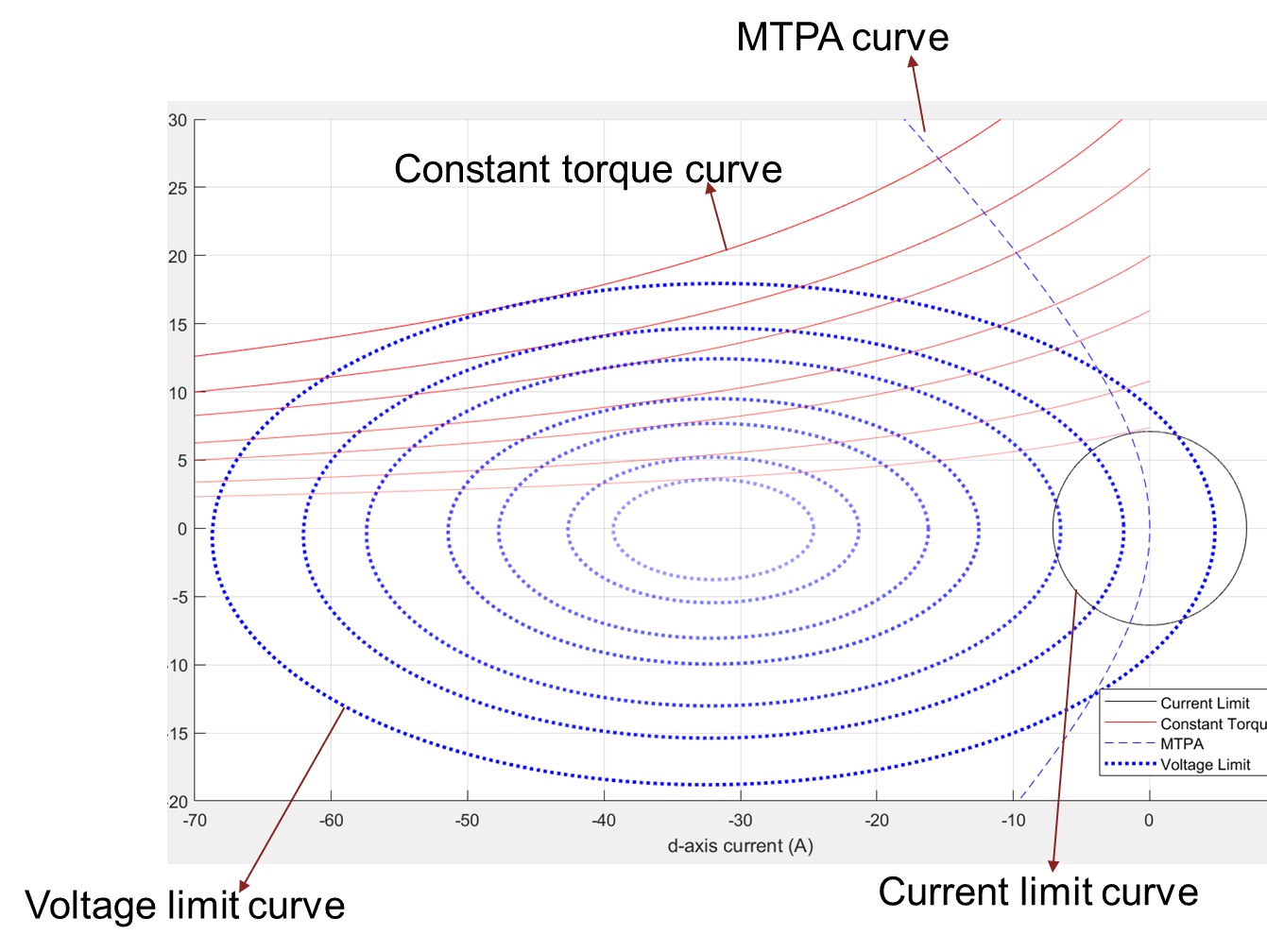Figure 12: Current limit circle, MTPA curve, a group of pairs of voltage limit curves and constant torque curves that tangentially touch the corresponding voltage limit curve, and MTPV curve.

### Using Constraint Curves

You can use the constraint curves in multiple ways. This section lists some of these methods.

### Find Maximum Operable Speed

You can find the maximum operable speed of a PMSM (SPMSM or IPMSM) in different ways. You can use the analytical equations to find the maximum speed. Using the constraint curves is another method. Note that you can use the machine for a limited speed due to several constraints (such as efficiency, mechanical stability, and maximum required speed).

From the constraint curves, you can obtain the maximum operable speed of a machine which is a point where the internal frictional torque (with no load torque) can be supported by the motor's produced torque. When the speed increases beyond the maximum speed, the frictional load torque increases and the motor fails to support the load torque.Figure 13 : Constraint curves of a PMSM, drawn at maximum operable speed, at 0 N.m. load torque.Figure 14: Zoomed-in portion from figure 13 to show the intersection of three curves - current limit circle, voltage limit ellipse, and constant torque curve.Figure 15: Drive characteristics of the motor that is marked with the operating points at the maximum speed.

The following code can be used to find the maximum speed of the motor.

```pmsm=mcb_SetPMSMMotorParameters('Teknic2310P'); pmsm.Rs=0.01; pmsm.Lq=pmsm.Ld*2; inverter=mcb_SetInverterParameters('BoostXL-DRV8305'); max_speed=mcbPMSMMaxSpeed(pmsm,inverter); disp('maximum speed is ' + string(max_speed) + ' rpm');```
```maximum speed is 6648 rpm ```

### Finding Corner Speed of Motor

The rated speed of a motor is the speed at which the motor can be loaded with the rated torque, while operating at rated voltage. This speed is also known as corner speed because at this speed, PMSM's speed-torque characteristics has a change of shape. For example, in figure 18, the point A marks the corner speed of the motor. This speed is also called the base speed. You can use analytical equations to find the corner speed. Using the constraint curves is another method.

From the constraint curves, you can determine the corner speed of a machine, which is a point where the voltage limit curve crosses the intersection of MTPA curve and the current limit circle. When the speed increases beyond the corner speed, the voltage limit curve does not enclose the intersection of MTPA curve and the current limit circle.Figure 16: Constraint curves of a PMSM drawn at base speed (corner speed), and at rated torque.Figure 17: Zoomed in portion of figure 16 to show the intersection of MTPA curve, current limit circle, and voltage limit curve.Figure 18: The speed-torque characteristics of a PMSM that is marked at corner speed at rated torque.

You can use the following code to find the corner speed of the PMSM:

```pmsm=mcb_SetPMSMMotorParameters('Teknic2310P'); pmsm.Rs=0.01; pmsm.Lq=pmsm.Ld*2; inverter=mcb_SetInverterParameters('BoostXL-DRV8305'); mcbPMSMSpeeds(pmsm,inverter,verbose=1);```
```Proceeding with the motorType as 'ipmsm'. Corner speed [rpm] is: 4905. Maximum speed [rpm] with VCLMT Field Weakening is: 6648. ```

### Identifying Operating Points (id,iq)

For a given speed and load torque, you can find the operating points of a machine. The following sections explain five selected operating points.

#### Case 1: MTPA Region of Operation

MTPA stands for Maximum Torque Per Ampere. A motor operates in MTPA region, when the ${v}_{s}=\sqrt{\left({v}_{d}^{2}+{v}_{q}^{2}\right)}$ is less than v_max.

To compute the optimal operating point, you can draw the constraint curves for the given speed and torque conditions.Figure 19: Constraint curves of a PMSM drawn at a torque of 0.1N.M. and at a speed of 5000rpm. The corner speed at the given torque is higher than the given speed, resulting in the possibility of MTPA operation.Figure 20: Zoomed-in portion of the figure 19 to show the selected operating point for the given speed and torque.

Any point along the portion of constant torque curve (between the marked points 1 and 2) that is within the region of overlap between the voltage limit ellipse and current limit circle results in the same torque output, while satisfying the voltage and current constraints. Point 1 results in the lowest current magnitude, and therefore, it is an optimal point of operation, which satisfies the voltage limit constraint and current limit constraint. In addition, this point meets the load torque demand and speed requirement.Figure 21 (a): Drive characteristics of a PMSM with the speed and torque point marked.

Figure 21 (b): Current curves of the PMSM for the loaded torque, with the operating point marked on them.

From the constraint curves shown in figure 20, you can notice that for the given speed, the voltage limit ellipse does not touch the operating point (id, iq), but encloses it. At a slightly higher speed (until the voltage limit curve touches the identified operating point) the same operating point (id, iq) is still the optimal operating point. Note that when the voltage limit curve touches the identified operating point (id, iq), ${v}_{s}$ is identical to ${v}_{\mathrm{max}}$, and the corresponding speed is the corner speed at the load torque. At a speed higher than this corner speed, the voltage limit is exceeded if you follow the MTPA trajectory, and therefore, you can start the field weakening (FW) operation.

You can use the following code to plot the figures.

```pmsm=mcb_SetPMSMMotorParameters('Teknic2310P'); pmsm.Rs=0.01; pmsm.Lq=pmsm.Ld*2; inverter=mcb_SetInverterParameters('BoostXL-DRV8305'); mcbPMSMCharacteristics(pmsm,inverter,torque=0.1,speed=5000,driveCharacteristics=0);```#### Case 2: Field-Weakening, Load Torque Lower Than Maximum Possible Torque at Given SpeedFigure 22: Constraint curve of the PMSM drawn at 0.1N.m. load torque and at 6000 rpm, with the constant torque curve passing through the intersection of current limit circle and voltage limit curve.

When you operate the motor at a speed higher than the corner speed along the MTPA trajectory, the voltage limit exceeds. Then the operating point moves along the constant torque curve to maintain the load torque demand. In figure 22, the constant torque curve passes through the intersection region of voltage limit curve and the current limit circle. This indicates that the when (id, iq) values are selected along the constant torque curve within this overlapping region, the load demand is satisfied. However, the extremes of the possible points are marked 1 and 2 in the figure. Point 1 results in the lowest current magnitude, and therefore, the most optimal point for operation while satisfying the voltage limit constraint, current limit constraint. It also meets the load torque demand and the speed requirement.Figure 23: Zoomed in portion of the figure 22 to show the selected operating point for optimal operation.Figure 24 (a): Drive characteristics of a PMSM with the speed and torque point marked.

Figure 24 (b): Current curves of the PMSM for the loaded torque, with the operating point marked on them.

You can use the following code to plot the figures.

```pmsm=mcb_SetPMSMMotorParameters('Teknic2310P'); pmsm.Rs=0.01; pmsm.Lq=pmsm.Ld*2; inverter=mcb_SetInverterParameters('BoostXL-DRV8305'); data=mcbPMSMCharacteristics(pmsm,inverter,torque=0.1,speed=6000,driveCharacteristics=0);```#### Case 3: Field Weakening, Load Torque More Than Maximum Possible Torque at Given Speed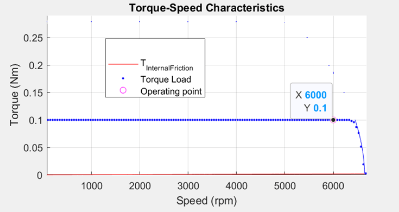Figure 25: Constraint curves of the PMSM drawn at 0.1 N.m. and 6000rpm speed, with the constant torque curve not passing through the intersection of current limit circle and voltage limit curve.

Figure 25 shows a situation when the demanded torque is higher than the possible torque at the given speed. In the figure, observe that the constant torque curve does not pass though the intersection region. Any point on the constant torque curve does not satisfy the current and voltage constraints. Therefore, only the maximum possible torque is returned as the operating point. This point is marked as 1 in the preceding figure.Figure 26: Zoomed in portion of the figure 25 showing the selected operating point.Figure 27 (a): Drive characteristics of a PMSM with the speed and torque point marked.

Figure 27 (b): Current curves of the PMSM for the loaded torque, with the operating point marked on them.

You can use the following code to plot the figures.

```pmsm=mcb_SetPMSMMotorParameters('Teknic2310P'); pmsm.Rs=0.01; pmsm.Lq=pmsm.Ld*2; inverter=mcb_SetInverterParameters('BoostXL-DRV8305'); mcbPMSMCharacteristics(pmsm,inverter,torque=0.1,speed=6575,driveCharacteristics=0);```#### Case 4: MTPV, Load Torque Less Than Maximum Possible Torque at Given SpeedFigure 28: Constraint curves of a PMSM (designed to have MTPV region of operation) drawn at 0.25N.m load and 20000rpm speed, with the constant torque curve passing through the intersection of current limit circle and voltage limit curve.

You can design a motor to have a possible MTPV operating region, by selecting a weaker magnet or by having a high current limit, or a combination of both. For the motors which have a possible MTPV operating region, the constraint curves are drawn in the preceding figure. For these motors, theoretical speed limit is infinite, but the motors' practical maximum achievable speed is limited by the frictional load.

For these motors, field weakening operation starts after the MTPA region of operation. Beyond a specific speed of operation, which can be called the MTPV initiation speed, the voltage ellipse shrinks further. At higher speeds, it converges approximately at the critical current point. To satisfy the load torque, you can pick any point along the constant torque curve, which also satisfies the voltage constraint and current constraints. That is, a point which lies within the intersection region of the current limit curve and the voltage limit curve.

The figure 28 shows a case where the demanded load torque is lower than a torque that the motor can provide at the operating speed. For such operation, the points 1 and 2 are marked as the extremes of the possible points along the constant torque curve that satisfy the voltage and current constraints. The point 1 has the lowest current magnitude, making it the optimal point of operation.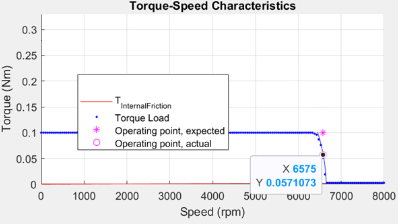Figure 29: Zoomed-in portion of figure 28 showing the selected operating point.Figure 30 (a): Drive characteristics of a PMSM with the speed and torque point marked (b) Current curves of the PMSM for the loaded torque, with the operating point marked on them.

You can use the following code to plot the figures.

```pmsm=mcb_SetPMSMMotorParameters('Teknic2310P'); pmsm.Rs=0.01; pmsm.I_rated=40; pmsm.B=9e-5; pmsm.Lq=pmsm.Ld*2; inverter=mcb_SetInverterParameters('BoostXL-DRV8305'); mcbPMSMCharacteristics(pmsm,inverter,torque=0.25,speed=20000,driveCharacteristics=0);```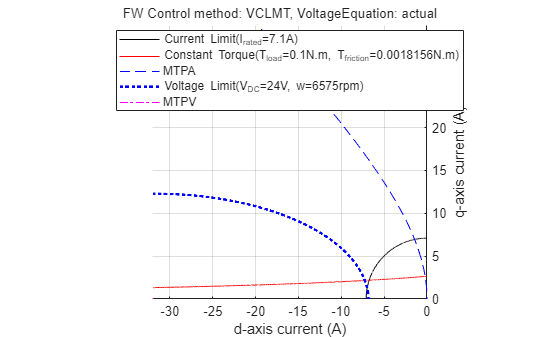#### Case 5: MTPV, Load Torque More Than Maximum Possible Torque at Given SpeedFigure 31: Constraint curve of a PMSM (designed to have MTPV region of operation) drawn at 0.25N.m load and 30000rpm speed, with the constant torque curve not passing through the intersection of current limit circle and voltage limit curve.

Sometimes, the load torque can be higher than the torque that the motor can provide at the operating speed. In the preceding figure, this is represented by the constant torque curve not passing through the intersection of the current limit curve and voltage limit curve. Under this condition, the maximum torque is picked from the voltage limit curve. This will be the point along the MTPV curve, intersecting the voltage limit curve.Figure 32: Zoomed in portion of the figure 31, showing the selected operating point for optimal operation.Figure 33 (a): Drive characteristics of a PMSM with the speed and torque point marked (b) Current curves of the PMSM for the loaded torque, with the operating point marked on them.

The preceding figure shows the selected operating point with circles. In addition, the speed-torque plot is also depicted with the expected torque point, which is higher than the possible torque at that speed.

```pmsm=mcb_SetPMSMMotorParameters('Teknic2310P'); pmsm.Rs=0.01; pmsm.I_rated=40; pmsm.B=9e-5; pmsm.Lq=pmsm.Ld*2; inverter=mcb_SetInverterParameters('BoostXL-DRV8305'); mcbPMSMCharacteristics(pmsm,inverter,torque=0.25,speed=30000,driveCharacteristics=0);```### Identifying Possible (MTPA-Field Weakening-MTPV) Control Currents

The preceding section explains that for different speeds and torques, optimal (id, iq) values can be derived.

In a typical motor control application, you need a reference current value for the operating speed and the reference torque. Such reference currents can be derived using the preceding methods, which result in optimal operations.

### LUT based PMSM Control Reference

The block "LUT based PMSM Control Reference" computes the id and iq look-up tables using the given motor parameters (either linear lumped parameters, or non-linear parameter maps) or uses the user-provided id, iq look-up table values.Figure 34 (a): LUT based PMSM Control Reference block, shipped as a part of MCB library. Figure 34 (b): The mask view for the block with options showing the input possibilities of motor parameters.

The LUT based PMSM Control reference block accepts multiple types of inputs that can utilize the externally generated (id, iq) LUTs or calculate the LUT based on the provided motor parameters (linear or non-linear). When the user selects the motor parameters (either linear or non-linear) as parameter input method, the LUT based PMSM Control Reference block computes the LUTs on the host before the start of simulation or before code-generation step.

### mcbGenerateTables

The function mcbGenerateTables ships with Motor Control Blockset™. You can use it to create id, iq LUTs on the host for custom grid sizes and grid point densities. The LUT based PMSM Control Reference block uses this function to compute the id, iq LUTs using the given motor parameters.

When the id, iq LUTs are generated, you can plot them in the d-q current space for multiple speed and torque values. When the point grid overlaps with the motor's constraint curves, you can obtain figure 36. In the figure, the dots at the intersection of the curves are only for representation purposes. The output figure window of the function will have segments of voltage limit curve and constant torque curves that are drawn within the current limit circle.Figure 35 (a): Constraint curves of a PMSM (designed to have MTPV operating region) overlapped with the grid of (id, iq) Look-up Tables for different speeds and load torques. Figure 35 (b): id LUT. Figure 35 (c): iq LUT.

You can use the following code to create and plot a grid of the id, iq with respect to speed and torque values.

```pmsm=mcb_SetPMSMMotorParameters('Teknic2310P'); pmsm.Rs=0.01; pmsm.I_rated=40; pmsm.B=9e-5; pmsm.Lq=pmsm.Ld*2; inverter=mcb_SetInverterParameters('BoostXL-DRV8305'); seed.drawLUTonConstraintCurves=1; seed.TBreakPointsCount=31; seed.wBreakPointsCount=32; PMSMLUT=mcbGenerateTables(pmsm,inverter,'idiqLUTs',seed);````disp(PMSMLUT)`
``` drawLUTonConstraintCurves: 1 TBreakPointsCount: 31 wBreakPointsCount: 32 trefVec: [-2.1646 -2.0203 -1.8760 -1.7317 -1.5874 -1.4431 -1.2988 -1.1545 -1.0101 -0.8658 -0.7215 -0.5772 -0.4329 -0.2886 -0.1443 0 0.1443 0.2886 0.4329 0.5772 0.7215 0.8658 1.0101 1.1545 1.2988 1.4431 1.5874 1.7317 1.8760 ... ] wrpmVec: [1 830 1660 2489 3318 4147 4977 5806 6635 7464 8294 9123 9952 10781 11611 12440 13269 14098 14928 15757 16586 17415 18245 19074 19903 20732 21562 22391 23220 24049 24879 25708] idTable: [31x32 double] iqTable: [31x32 double] ```

### Simulation Using id, iq LUTsFigure 36: View of Simulink model that is created to use the LUT based PMSM Control Reference block to generate [id, iq] reference values for a given load torque and a range of speed values. This model has been used to create the (id, iq) reference values for fractional rated torque values for different speeds drawn in the figures 21, 24, 27, 30, and 33.

As you can see, the LUT based PMSM Control Reference block can be used with a given load torque and a range of speed values to derive the possible speed-torque characteristic curve, similar to the ones shown in the figures in the earlier sections.

The following figures are constraint curves of the motor that overlaps with the data from the simulations using the LUT based PMSM Control Reference block.Figure 37 (a): Constraint curves of a PMSM drawn at envelope torque, showing the overlapped (id, iq) reference values generated from the LUT based PMSM Control Reference block.

Figure 37 (b): Constraint curves of a PMSM drawn at half the rated torque, showing the overlapped [id, iq] reference values generated from the LUT based PMSM Control Reference block.

From the figures 37(a) and 37(b), notice that the black stars are the contour of points derived from the simulation that overlaps the corresponding constraint curves. For both these cases, the MTPA region is not shown, because the torque demand starts from the maximum, and does not increase. For the case of 100% load, you can see that the operating points follow the field weakening path along the current constraint curve and then the MTPV curve. For the case of 50%, you can see that the operating points follow the constant torque curve and then the MTPV curve.

An example mcb_pmsm_nonlin_fwc.slx uses the non-linear data from FEA simulation software to demonstrate usage of non-linear motor parameters to derive the id, iq LUTs and derives the reference currents based on the operating conditions.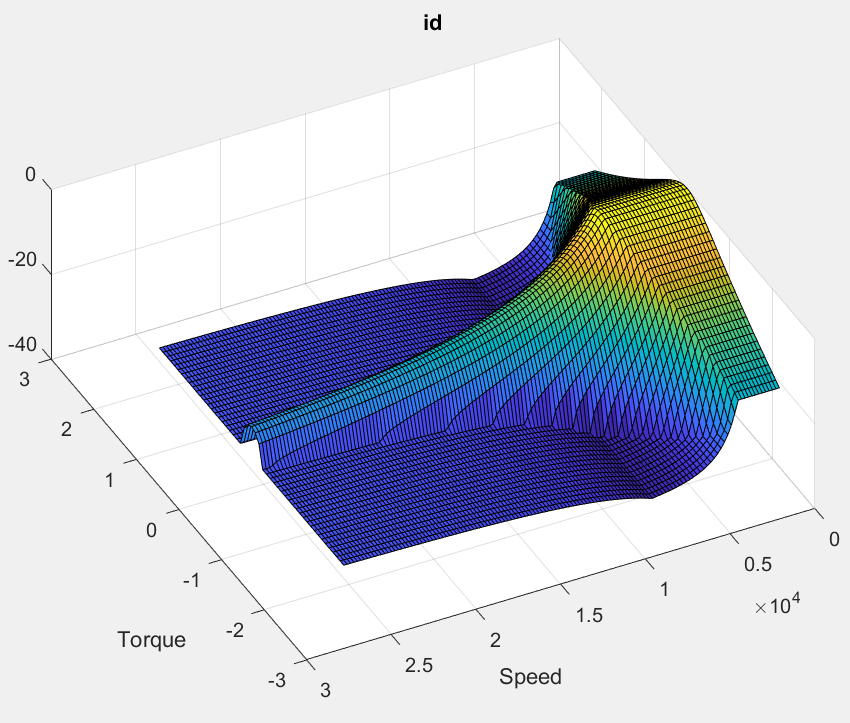Figure 38: The example model mcb_pmsm_nonlin_fwc.slx.